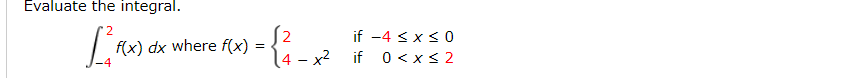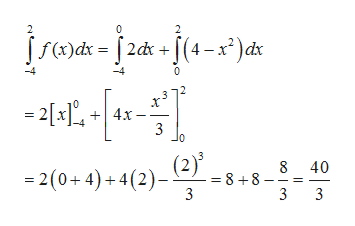# Evaluate the integral.if -4 < x < 0f(x) dx where f(x)0 < x< 24 - x2if

Question
4 views

Evaluate The Integralhelp_outlineImage TranscriptioncloseEvaluate the integral. if -4 < x < 0 f(x) dx where f(x) 0 < x< 2 4 - x2 if fullscreen
check_circle

Step 1

Given function is

Step 2

By using the property of definit integral , we have

Step 3

Now, we substitute f(x) and t...help_outlineImage TranscriptioncloseS(4-x²)dx [ f(x)dx |2dx = 2[x], +| 4x- 3 (2)' 8 40 = 2(0+ 4) + 4(2)- = 8 +8- 3 3 fullscreen

### Want to see the full answer?

See Solution

#### Want to see this answer and more?

Solutions are written by subject experts who are available 24/7. Questions are typically answered within 1 hour.*

See Solution
*Response times may vary by subject and question.
Tagged in

### Integration